# Test Prep Plan - Take a practice test

Take this practice test to check your existing knowledge of the course material. We'll review your answers and create a Test Prep Plan for you based on your results.
How Test Prep Plans work
1
2Based on your results, we'll create a customized Test Prep Plan just for you!
3Study smarter
Study more effectively: skip concepts you already know and focus on what you still need to learn.

# Properties of Definite Integrals Chapter Exam

Exam Instructions:

Choose your answers to the questions and click 'Next' to see the next set of questions. You can skip questions if you would like and come back to them later with the yellow "Go To First Skipped Question" button. When you have completed the practice exam, a green submit button will appear. Click it to see your results. Good luck!

### Page 1

#### Question 1 1. Given the following information, what does z equal?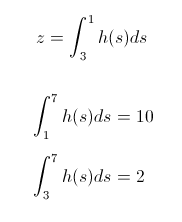#### Question 2 2. How many terms are in the Riemann sum represented below?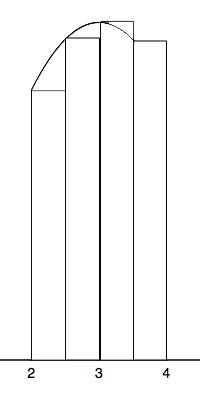#### Question 3 3. Which integral below is equal to the following integral?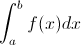#### Question 4 4. Which of the following integrals is equal to the following summation over the continuous region x=a to x=b?#### Question 5 5. In the following integral, g(x) is defined as which of the following?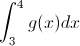### Page 2

#### Question 7 7. You are going to take a Riemann Sum of the area below. To do this, you divide the region into four slices of equal width. How wide is each slice?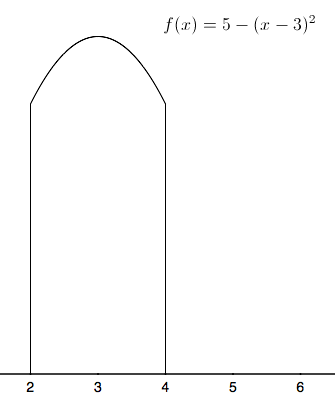#### Question 8 8. Given f(x) on the graph below, which of the following integrals will equal 0?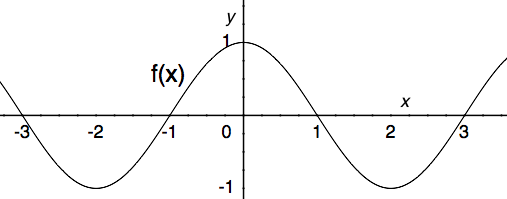#### Question 9 9. Which integral below is equal to the following integral?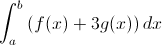#### Question 10 10. Evaluate the following.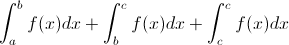### Page 3

#### Question 11 11. Estimate the area beneath the curve f(x) using the two term Riemann sum as shown below.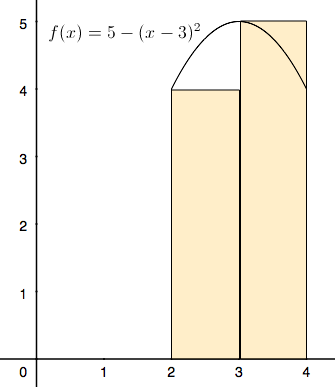#### Question 12 12. Given f(x) on the graph, what does z equal?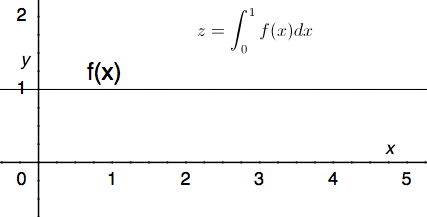#### Question 13 13. The following integral can be re-written as: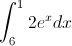#### Question 14 14. Which of the following integrals represents the area under the graph below?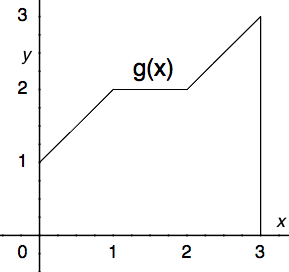#### Question 15 15. Which of the following represents the area of the FIRST rectangle being used to estimate the area under the curve f(x) between x = 0 and x = 2 when divided into 4 slices?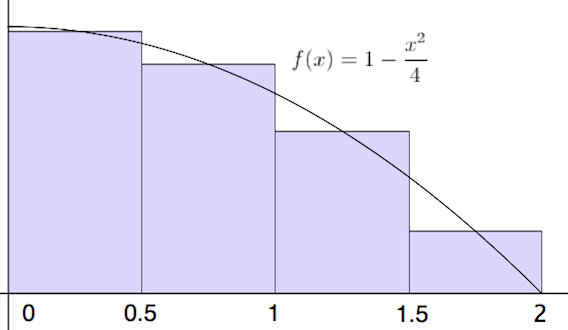### Page 4

#### Question 16 16. Using the single term Riemann sum as shown, what is the area beneath the curve f(x) between 0 and two pi?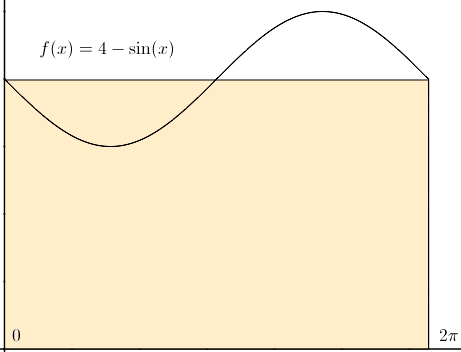#### Question 17 17. In the following integral, 4 is defined as which of the following?#### Question 18 18. Write out the first four terms of a Riemann sum equivalent to the following integral.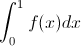#### Question 19 19. Which of the following integrals is equal to the following summation over the continuous region x=a to x=b?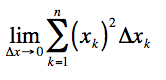#### Question 20 20. Which of the following graphs shows the correct region of integration for the integral below?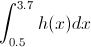#### Properties of Definite Integrals Chapter Exam Instructions

Choose your answers to the questions and click 'Next' to see the next set of questions. You can skip questions if you would like and come back to them later with the yellow "Go To First Skipped Question" button. When you have completed the practice exam, a green submit button will appear. Click it to see your results. Good luck!

Support